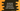# JavaScript parseFloat method explanation with examples## JavaScript parseFloat method explanation with examples:

The parseFloat method is an inbuilt method and we can use it to convert a string argument value to floating-point value. In this post, we will learn how to use parseFloat method with different examples.

### Definition of parseFloat:

The parseFloat method is defined as like below:

``parseFloat(v)``

It takes one parameter, v, which is the value to convert or parse. It converts the string to a number and returns that. If it can’t convert the value, it returns NaN.

It parses the first number found in a string. If there are any leading or trailing spaces, it ignores.

### parseFloat return value:

This method returns the number it converts. It returns NaN if it doesn’t find any number.

### Example of parseFloat:

Let’s take an example of parseFloat:

``````let arrayOfStrings = [
"12",
"12.34",
"-12.34",
"0",
"NaN",
"",
"   12.34   ",
"hello",
"123hello",
];

arrayOfStrings.forEach((i) => console.log(`\${i} => \${parseFloat(i)}`));``````

If you run this program, it will print:

``````12 => 12
12.34 => 12.34
-12.34 => -12.34
0 => 0
NaN => NaN
=> NaN
12.34    => 12.34
hello => NaN
123hello => 123``````

Here,

• arrayOfStrings is an array of strings.
• By using forEach, we are iterating through the elements of the array one by one. For each element, it prints the value of parseFloat.
• For “12” string, it is number 12
• For “12.34” string, it is number 12.34
• For “-12.34” string, it is number -12.34
• For “0” string, it is number 0
• For “NaN” string, it is NaN
• For "" string, it is NaN
• For ” 12.34 ” string, it is number 12.34. It ignored the leading and trailing strings.
• For “hello” string, it is NaN because “hello” is not a number.
• For “123hello” string, it is 123 because “123” is the leading number in the string.

### Browser support of parseFloat:

The parseFloat method is an ECMAScript1 or ES1 feature. It is available in all browsers.